Trial ends in

# Calibration Procedures for Orthogonal Superposition Rheology

doi: 10.3791/61965 Published: November 18, 2020
Ran Tao1,2, Aaron M. Forster1

### Abstract

Orthogonal superposition (OSP) rheology is an advanced rheological technique that involves superimposing a small-amplitude oscillatory shear deformation orthogonal to a primary shear flow. This technique allows the measurement of structural dynamics of complex fluids under non-linear flow conditions, which is important for the understanding and prediction of the performance of a wide range of complex fluids. The OSP rheological technique has a long history of development since the 1960s, mainly through the custom-built devices that highlighted the power of this technique. The OSP technique is now commercially available to the rheology community. Given the complicated design of the OSP geometry and the non-ideal flow field, users should understand the magnitude and sources of measurement error. This study presents calibration procedures using Newtonian fluids that includes recommendations for best practices to reduce measurement errors. Specifically, detailed information on the end-effect factor determination method, sample filling procedure, and identification of the appropriate measurement range (e.g., shear rate, frequency, etc.) are provided.

### Introduction

Understanding the rheological properties of complex fluids is essential to many industries for the development and manufacture of reliable and reproducible products1. These “complex fluids” include suspensions, polymeric liquids, and foams that widely exist in our everyday life, for example, in personal care products, foods, cosmetics, and household products. The rheological or flow properties (e.g., viscosity) are key quantities of interest in establishing performance metrics for end use and processability, but flow properties are interconnected with the microstructures that exist within complex fluids. One prominent characteristic of complex fluids that distinguishes them from simple liquids is that they possess diverse microstructures spanning multiple length scales2. Those microstructures can be easily affected by different flow conditions, which, in turn, result in changes in their macroscopic properties. Unlocking this structure-property loop via non-linear viscoelastic behavior of complex fluids in response to flow and deformation remains a challenging task for experimental rheologists.

Orthogonal superposition (OSP) rheology3 is a robust technique to address this measurement challenge. In this technique, a small amplitude oscillatory shear flow is superimposed orthogonally to a unidirectional primary steady-shear flow, which enables the simultaneous measurement of a viscoelastic relaxation spectrum under the imposed primary shear flow. To be more specific, the small oscillatory shear perturbation can be analyzed using theories in linear viscoelasticity4, while the non-linear flow condition is achieved by the primary steady-shear flow. As the two flow fields are orthogonal and thus not coupled, the perturbation spectra can be directly related to the variation of the microstructure under the primary non-linear flow5. This advanced measurement technique offers an opportunity to elucidate structure-property-processing relationships in complex fluids to optimize their formulation, processing, and application.

The implementation of modern OSP rheology was not the result of a sudden epiphany; rather, it is based on many decades of development of custom devices. The first custom made OSP apparatus is dated back to 1966 by Simmons6, and many efforts were made thereafter7,8,9,10. Those early custom-built devices suffer from many drawbacks such as alignment issues, the pumping flow effect (due to the axial movement of the bob to provide orthogonal oscillation), and limits to instrument sensitivity. In 1997, Vermant et al.3 modified the force rebalance transducer (FRT) on a commercial separate motor-transducer rheometer, which enabled OSP measurements for fluids with a wider viscosity range than previous devices. This modification enables the normal force rebalance transducer to function as a stress-controlled rheometer, imposing an axial oscillation in addition to a measurement of the axial force. Recently, the geometries required for OSP measurements, after the methodology by Vermant, have been released for a commercial separate motor-transducer rheometer.

Since the advent of commercial OSP rheology, there is a growing interest in applying this technique for the investigation of various complex fluids. Examples include colloidal suspensions11,12, colloidal gels13,14 and glasses15,16,17. While the availability of the commercial instrument promotes OSP research, the complicated OSP geometry requires a deeper understanding of the measurement than other routine rheological techniques. The OSP flow cell is based on a double-wall concentric cylinder (or Couette) geometry. It features an open top and open bottom design to enable fluid to flow back and forth between the annular gaps and the reservoir. Despite the optimization made to the geometry design by the manufacturer, when undergoing OSP operation the fluid experiences an inhomogeneous flow field, geometric end effects, and residual pumping flow, all of which can introduce substantial experimental error. Our previous work18 reported important end-effect correction procedures using Newtonian fluids for this technique. To obtain correct viscosity results, appropriate end-effect factors in both primary and orthogonal directions should be applied. In this protocol, we aim to present a detailed calibration methodology for the OSP rheological technique and provide recommendations for best practices to reduce measurement errors. The procedures delineated in this paper on OSP geometry setup, sample loading, and OSP test settings should be easily adoptable and translated for non-Newtonian fluids measurements. We advise that users utilize the calibration procedures described here to determine the end-effect correction factors for their applications prior to OSP measurements on any fluid classification (Newtonian or Non-Newtonian). We note that the calibration procedures for end factors have not been reported previously. The protocol provided in the present paper also describes step-by-step guide and tips on how to perform accurate rheological measurements in general and the technical resource on the understanding of “raw” data versus “measured” data, which may be overlooked by rheometer users.

### Protocol

1. Rheometer setup

NOTE: The protocol in this section describes basic steps to run a rheology experiment (for either a separate motor-transducer rheometer or a combined motor-transducer rheometer), including preparation of the setup, installation of appropriate geometry, loading the test material, setting up the experiment procedure, specifying the geometry, and starting the test. Specific instructions and notes are provided for OSP operation. To minimize thermal gradients in the transducer, it is recommended to power the rheometer for at least 30 min prior to the operation. The rheometer software used in this protocol for instrument control and data collection are noted in the Table of Materials. See Table 1 for rheometer specifications.

1. Before setting up the rheometer, enable the Orthogonal Superposition feature in the rheometer software. Install a lower platinum resistance-thermometer (PRT) on the test station for temperature measurement and an environmental control device.
NOTE: Lift the stage to maximum height for the installation process (Figure 1a). Install proper PRT before mounting the environmental control device. Be careful to not hit the PRT with the environmental control device during installation. Use the supplied spanner wrench to secure the environmental control device on the test station.
2. Install the double wall concentric cylinder geometry.
1. Assemble the inner and outer cylinders (Figure 1b) properly to complete the double-wall cup configuration.
NOTE: Before assembling the cup, check the O-ring condition (for cracks, swelling or other damage) on the inner cylinder and replace if needed.
2. Insert the cup into the environmental control device and align the geometry properly.
3. Press the lower geometry (cup) downward to compress the spring-loaded PRT while tightening the thumbscrew using a torque screwdriver (0.56 N m fixed).
NOTE: To check if the lower geometry is correctly installed, disable the motor power, and use a finger to spin the geometry. If the lower geometry spins freely in the environment control device, then it is installed properly and continue the next step. If it does not spin freely, remove the components from the test station in reverse order of the previous steps and then re-install the lower geometry. Verify that the temperature signal is received from the lower PRT. The rheometer should automatically recognize the temperature sensor by default; if not, select the lower PRT as the Temperature Control Sensor source in the temperature control options from the rheometer software.
4. Install the upper geometry (bob) on the transducer shaft. Tare the normal force and torque by clicking the Tare Transducer button in the transducer control panel from the rheometer software or using Tare Torque and Tare Normal on the Instrument tab from the instrument touch screen. A picture of the complete rheometer setup is shown in Figure 1c.
5. Zero the gap between the upper and lower geometries by clicking the Zero Fixture button in the gap control panel from either the rheometer software or from the instrument touch screen. Perform geometry mass calibration if needed.
NOTE: Check the geometry documentation supplied by the manufacturer to see if the upper tool mass value is available. If not, perform geometry mass calibration at the end of this step. Follow the on-screen instructions to perform the upper tool mass calibration. Upon completion, confirm that the correct new fixture mass is accepted.

1. Lift the stage to provide enough workspace to load the test material into the cup.
2. Use a pipette or a spatula to load the test material into the cup. Carefully handle the test material to minimize entrainment of air into the fluid.
3. Lower the bob into the cup to the geometry gap set point and lifted out to determine the fluid level in the loaded geometry. The goal is to achieve a fluid contact line that is slightly (approximately 2 mm) above the lower edge of the bob upper opening.
NOTE: This process may require lengthy wait times to reach the desired fluid level because of the small annular gap width of the geometry and the relatively large volume of sample needed. The wait times mainly depend on the viscosity of the test material. For example, a highly viscous liquid takes longer to flow into the gaps between the cylinders and completely wet the bob surfaces.
4. Lower the upper geometry carefully into the fluid to reach the geometry gap set point of 8 mm. This process is illustrated as Step 1 in Figure 2c. Wait a few minutes while the bob is being kept at position (iii) where the gap is set to 8 mm.
NOTE: When the bob end surface contacts the fluid, reduce the downward velocity of the bob. For a high viscosity liquid or yield-stress fluid, closely monitor the normal force readings to prevent the transducer from overloading during this process.
5. Lift the bob vertically using the slow slew speed of the instrument to a position where the wetted fluid contact line can be visually inspected (Figure 3). The contact line indicates the fluid level in the geometry at the gap set point. If the line on the bob is below the upper end of the bob (lower rim of the upper opening on the bob), it indicates that the fluid height is lower than the inner cylinder height and additional test material should be added to the geometry.
6. Carefully lift the bob to the previous loading position to allow for enough workspace (Step 2 in Figure 2c) and load an additional amount of test material into the cup as needed. Slowly move the bob up or down to avoid cavitation. Add the test material carefully to prevent introducing additional air bubbles.
7. Lower the upper geometry into the fluid and set to the final geometry gap again. Repeat Steps 1 and 2 (Figure 2c) until the wetted contact line on the bob is approximately 2 mm above the lower rim of the upper bob opening as shown in Figure 3a. Also check that the lower rim of the upper opening on the bob is properly wetted (Figure 3b). Move the bob to the geometry gap set point and allow the test material to relax.
NOTE: The wait time depends on the viscosity of the standard material. For example, for a 1 Pa s liquid, a wait time of 15 min is sufficient; whereas for a 100 Pa s liquid, a much longer wait time (4 h) is needed. This process is illustrated as Step 3 in Figure 2c. The complete sample loading procedure is illustrated in Figure 2. High viscosity fluids require extended time and are difficult to load. To reduce the wait time, increasing the temperature by a few degrees may be helpful to facilitate the viscous calibration liquid to flow.

3. Running viscosity calibration measurements

NOTE: The calibration protocols provided in this paper are specific to the end-effect factors applied for the OSP technique. This does not include routine calibrations or verification checks, including torque and normal force calibrations, phase angle check, PDMS check, etc. that are recommended by individual rheometer manufacturers. Those procedures should be performed prior to the calibration protocols in the present paper. The readers should refer to the User Manual of the rheometer manufacturer for the procedures of performing routine calibrations or checks. The silicone viscosity standards used in this protocol are noted in the Table of Materials.

1. Specify the geometry
NOTE: Before setting up the experiment, make sure the correct geometry is selected in the rheometer software. For first-time use, create a new orthogonal double wall concentric cylinder geometry in the rheometer software following the steps below.
1. Add a new orthogonal double wall concentric cylinder geometry.
2. Enter the dimensions for the geometry as shown in Table 2.
NOTE: The numbers and their corresponding symbols are inscribed on the bob and cup. The operating gap is 8 mm for the experimental geometry used here but should be specified by the manufacturer. Therefore, the inner cylinder height is equal to (immersed height + 8 mm).
2. Specify the geometry constants. Fill in the fields of geometry inertia and geometry mass with proper values. Enter 1.00 for both the end-effect factor and orthogonal end-effect factor.
NOTE: The geometry inertia for the 0.5 mm and 1.0 mm gap OSP geometries specified by the manufacturer are 15.5 µN m s2 and 10.3 µN m s2, respectively. Make sure that the correct value for the upper geometry mass is entered. This value can be found in the geometry documentation provided by the manufacturer. Alternatively, perform geometry mass calibration under the geometry calibration tab (Protocol step 1.2.5) and confirm that the correct new fixture mass is applied. The default end-effect factor (CL) is 1.065 and the orthogonal end-effect factor (CLo) is 1.04. Change both fields to 1.00. The stress constants are automatically calculated from the dimensions and end-effect factors. The strain constants are determined by the geometry dimensions only (expressions are provided in previous work18). The definitions of the dimensions are described in Table 2 and indicated in Figure 4. The expressions for the (primary) stress constant, Kτ, and orthogonal (linear) stress constant, Kτο, are: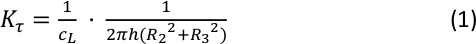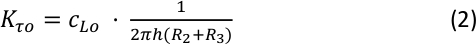4. Steady shear rate sweep tests

NOTE: Viscosity calibration measurements are performed independently in either the primary direction or the orthogonal direction to calibrate CL or CLo. For the primary direction, steady shear viscosity is measured by performing shear rate sweep tests. For the orthogonal direction, dynamic complex viscosity is measured by performing orthogonal frequency sweep tests.

1. Condition the sample at 25 °C for 15 min to allow the test material to reach thermal equilibrium.
NOTE: The calibration measurements are performed at the temperature at which the certified viscosity of the standard liquid is reported, i.e., 25 °C. The readers may use a different test temperature suitable for their Newtonian standard liquids. An equilibration time or soak time, i.e., 15 min, is recommended to ensure that the environmental control device, geometries, and sample to reach thermal equilibrium.
2. Select the Flow Sweep Test under the Experiment Procedure in the rheometer software. Set the test temperature to 25 °C under Environment Control.
3. Specify the shear rate range from 0.01 s−1 to 100.0 s−1 with data recording at 10 points per decade logarithmically. Enable automatic steady-state determination.
NOTE: The shear rate range used here is based on the instrument torque sensitivity limits (Table 1) and the measuring liquid. For example, for a higher viscosity liquid (e.g., 300 Pa s), a lower shear rate range of 10−4 s−1 to 1 s−1 may be used, and vice versa.
4. Start the experiment from the rheometer software.

5. Orthogonal frequency sweep tests

1. Set the normal force transducer to FRT mode from the transducer control panel in the rheometer software.
NOTE: The default transducer setting for the normal force transducer is spring mode for this separate motor-transducer rheometer. In the OSP operation, the normal force transducer operates as a stress-controlled or a combined motor-transducer rheometer to apply axial deformation while measuring the axial force simultaneously. The normal force transducer must be set in FRT mode to perform OSP tests.
2. Condition the sample at 25 °C for 15 min to ensure thermal equilibration.
3. Select the Orthogonal Frequency Sweep test under the Experiment Procedure in the rheometer software. Set the test temperature to 25 °C.
4. Specify the desired normal strain and enter 0.0 s−1 for the shear rate in the rotational direction.
NOTE: The maximum normal strain (axial strain amplitude) is dependent on the gap width of the OSP geometry and is limited by the maximum orthogonal oscillation displacement of the rheometer, i.e., 50 µm (Table 1).
5. Specify the angular frequency range from 0.1 to 40 rad/s at 10 points per decade logarithmically.
NOTE: The angular frequency range used here is a recommended range for OSP operation based on the instrument axial frequency sensitivity limits (Table 1) and the consideration of gap loading conditions18. See the Discussion section for more details.
6. Start the experiment from the rheometer software.

6. Performing analysis

1. Determination of the primary end-effect factor
1. Export the steady shear rate sweep results (from Protocol step 4.4.) into an open file format such as .csv or .txt.
2. Calculate the average value of the reported viscosities over the appropriate shear rate range in a spreadsheet software.
NOTE: Only the viscosity data with corresponding torque values above the manufacture specified limits are used to calculate the average viscosity. The averaged viscosity value is defined as the uncorrected primary viscosity.
3. Find the primary end-effect factor using the averaged viscosity value.
NOTE: This section is provided here to show the derivation of the relationship between the primary end effect factor and the direct viscosity output from the rheometer software. An example of the calculation of the end factor from the experimental data is demonstrated in the Representative Results section. The primary steady shear viscosity is the ratio of shear stress τ to the shear rate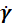, which is calculated from the raw signals of torque M and rotational velocity Ω via the geometry constants (Kτ and Kγ). The expression is given by: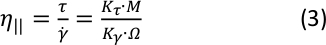where Kτ is the primary stress constant (Equation 1) and Kγ is the primary strain constant which is solely dependent on the geometric dimensions. Therefore, substituting Equation 1 into Equation 3, the calculated primary viscosity, or the output viscosity values from the rheometer software, is shown to be inversely proportional to the primary end-effect factor CL (note that all other variables in Equation 3 are either geometric constants or raw measurement signals):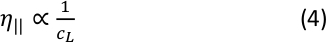Note that Equation 3 is a general expression to any rotational rheometry where the measured viscosity is calculated from the raw data, i.e., torque and velocity, via the stress and strain constants that depend on different geometry used, e.g., cone-plate, parallel plate, concentric cylinder, etc.

7. Determination of the orthogonal end-effect factor

1. Export the orthogonal frequency sweep results (from Protocol step 5.6.) into an open file format such as .csv or .txt.
2. Calculate the average value of the reported OSP complex viscosity over the appropriate angular frequency range in a spreadsheet software.
NOTE: Only the viscosity data with corresponding oscillation force values above the manufacturer specified limits are used to calculate the average viscosity. The averaged viscosity value is defined as the uncorrected orthogonal complex viscosity.
3. Find the orthogonal end-effect factor using the averaged complex viscosity value.
NOTE: This section is provided here to show the derivation of the relationship between the orthogonal end effect factor and the orthogonal complex viscosity output from the rheometer software. An example of the calculation of the orthogonal end factor from the experimental data is demonstrated in the Representative Results section. The orthogonal complex viscosity equals the orthogonal complex shear modulus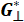divided by the orthogonal oscillatory frequency ω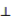, which can be expressed as the equation below through the oscillation force F, oscillation displacement θ, frequency ω(all three of which are raw signals), and the geometry constants (Kτο and Kγο):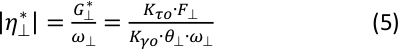where Kτο is the orthogonal stress constant (Equation 2) and Kγο is the orthogonal strain constant, which is solely related to the geometric dimensions. Therefore, substituting Equation 2 into Equation 5, the calculated orthogonal complex viscosity, or the output OSP complex viscosity values from the rheometer software, is shown to be directly proportional to the orthogonal end-effect factor CLo (note that all other variables in Equation 5 are either geometric constants or raw measurement signals):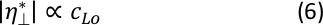Note that Equation 5 is a general expression for any linear motion measurements where the measured complex viscosity is calculated from the raw data, i.e., force, displacement, and frequency, via the stress and strain constants that depend on the geometry used, e.g., cone-plate, parallel plate, concentric cylinder, etc.

8. Viscosity verification check by OSP measurements

NOTE: This step is to verify if the corrections are valid using the calibrated end-effect factors obtained from the calibration experiments.

1. Enter the calibrated values for the end-effect factor and orthogonal end-effect factor under the geometry constants, initially these values were set equal to 1.00. The stress constants are automatically updated, and the values are as shown in Table 3.
2. Set up a same experimental procedure following the steps in the orthogonal frequency sweep tests. Enter 1.0 s−1 for the shear rate.
3. Start the experiment.

### Representative Results

Representative results from the viscosity calibration measurements on a 12.2 Pa s silicone viscosity standard are represented in Figure 5 and Figure 6. Note that the primary end-effect factor and the orthogonal end-effect factor are both set to 1.00 for the calibration runs. Figure 5 shows the steady shear viscosity and the torque as a function of shear rate on a double y-axis plot. The silicone liquid is a Newtonian fluid; as expected, a constant viscosity independent of the applied shear rate is obtained. The measured torque increases linearly as shear rate increases, and all of the data are above the low torque limit, 0.1 µN m, according to the manufacturer’ specifications (Table 1). Therefore, all the viscosity data in Figure 5 are used to calculate the average value, i.e., 14.3 Pa s (ηuncorr). Note that this uncorrected viscosity value is higher than the actual viscosity, i.e., 12.2 Pa s (ηcorr), as shown by the solid line in Figure 5, by 17 %. According to Equation 4, the primary viscosity is inversely proportional to CL, so the new CL that should be applied to obtain the correct viscosity is: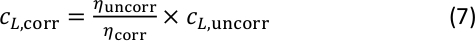Therefore, the correct primary end-effect factor CL is equal to 14.3 Pa s divided by 12.2 Pa s (CL,uncorr = 1.00) that equals 1.17.

Figure 6 shows the results from the orthogonal frequency sweep tests at different orthogonal strain amplitudes from 0.5 % to 9.4 % for the 12.2 Pa s viscosity standard. A Newtonian response is observed, as shown by the constant orthogonal complex viscosity with varying frequency. Similarly to the primary viscosity, without correction (CLo,uncorr = 1), the measured orthogonal complex viscosity overestimates the actual viscosity of 12.2 Pa s (ηcorr), as depicted by the solid line. All the viscosity data at different strains coincide with each other, indicating that the applied strains are in the linear range. The measured oscillation force plotted on the right y-axis, increases linearly with increasing frequency (Equation 5). The dashed line in Figure 6 represents the lower limit of the axial oscillation force for the transducer, i.e., 0.001 N (Table 1). Only the viscosity data with corresponding orthogonal force values above this sensitivity level are used to calculate the average viscosity for correction. The averaged orthogonal complex viscosity is 15.4 Pa s (ηuncorr), which is 26 % higher than the actual viscosity. According to Equation 6, the orthogonal complex viscosity is proportional to CLo, so the expression for the new CLo is: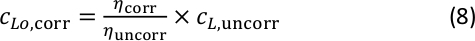Therefore, the correct orthogonal end-effect factor CLo is equal to 12.2 Pa s divided by 15.4 Pa s (CLo,uncorr = 1.00) that equals 0.79.

After obtaining the calibrated values for CL and CLo, it is recommended to run a verification test by performing an orthogonal superposition measurement under steady shear. Compared to the calibration measurements, which used only primary or oscillatory shear, both flow modes are employed simultaneously. The steady shear viscosity and orthogonal complex viscosity are measured from a single test, and the results are shown in Figure 7. Also plotted in the figure are the orthogonal oscillation force on the right y-axis. Only the data with values greater than the instrument force resolution are plotted. Since the correct end-effect factors are applied (Table 3), the measured viscosities in both directions match the accepted oil viscosity value of 12.2 Pa s. This graph can be generated by adding those outputs as plotting variables and displaying in the rheometer software for a quick check of the calibration procedure.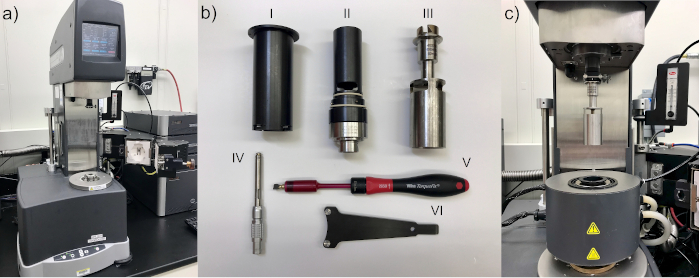Figure 1: Pictures of the rheometer, the OSP geometry, and the Advanced Peltier System (APS). (a) Rheometer test station. (b) Components of the orthogonal double wall concentric cylinder geometry: the outer cylinder (I), inner cylinder (II), and the center cylinder or bob (III); the PRT (IV), the torque screwdriver (V), and the spanner wrench (VI). See Table of Materials for the part number. The PRT, torque screwdriver, and spanner wrench are included in the APS kit. (c) The rheometer setup after the installation of the environmental control device and the orthogonal double wall concentric cylinder geometry for experiments. Please click here to view a larger version of this figure.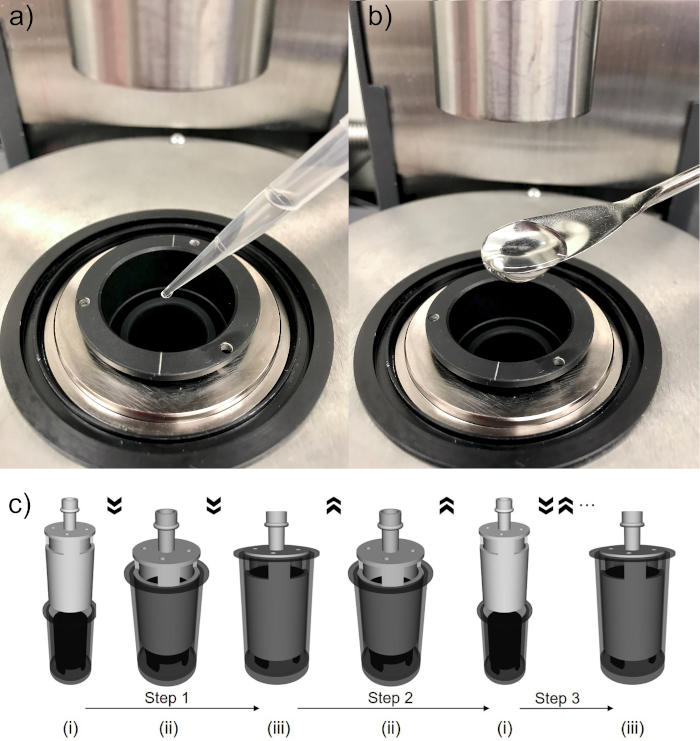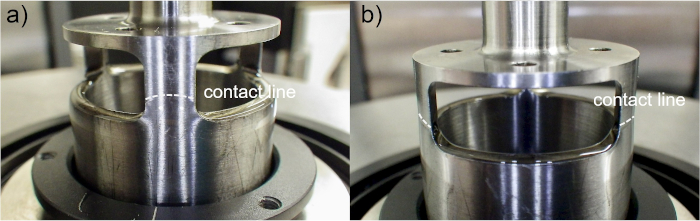Figure 3: Visual inspection of the wetted fluid contact line on the bob after lifting the bob out of the double wall cup. (a) Front view showing the contact line slightly above the upper bob end. (b) Side view showing the lower rim of the upper openings on the bob is properly wetted. The white dashed lines indicate the wetted fluid contact line on the bob. Please click here to view a larger version of this figure.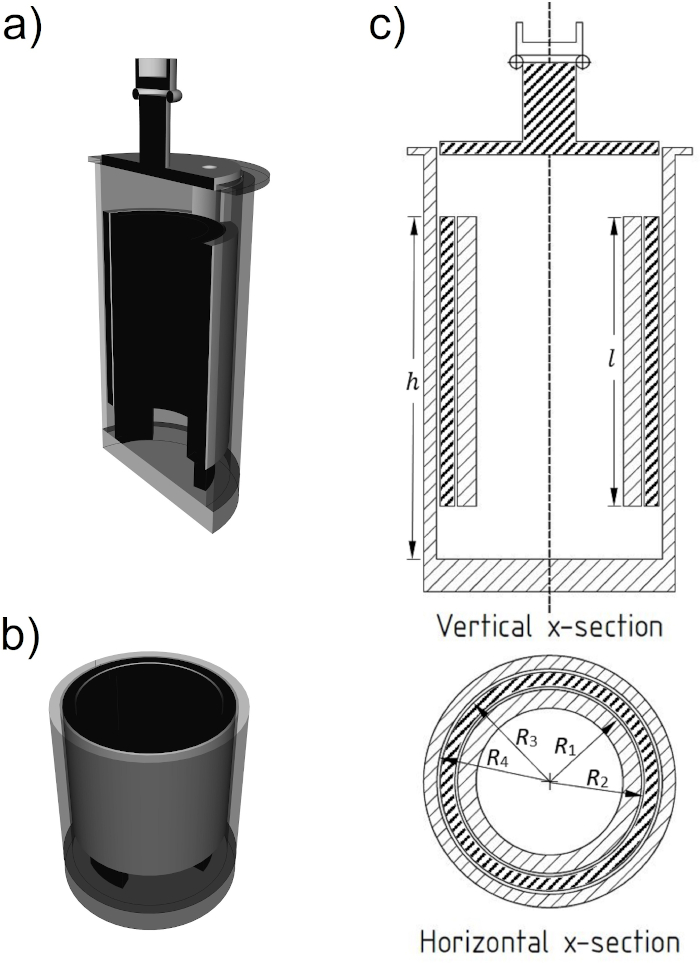Figure 4: Schematic representations of the vertical and horizontal cross sections of OSP double-wall concentric cylinder geometry. (a) Vertical cross section in a 3D view. (b) Horizontal cross section in a 3D view. (c) 2D layout of the geometry indicating the dimensions (Table 1).  Please click here to view a larger version of this figure.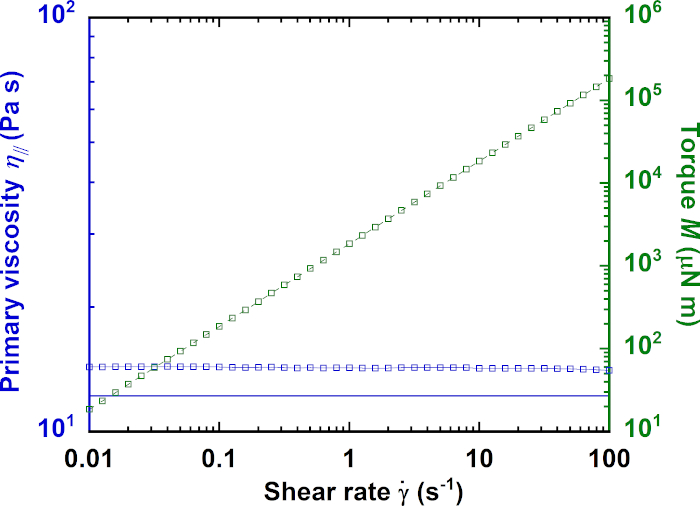Figure 5: Results from steady-shear rate sweep tests on a 12.2 Pa s viscosity standard. The primary steady shear viscosity (left y-axis) and torque (right y-axis) are shown as a function of shear rate. The solid line represents the actual viscosity of the silicone fluid. Please click here to view a larger version of this figure.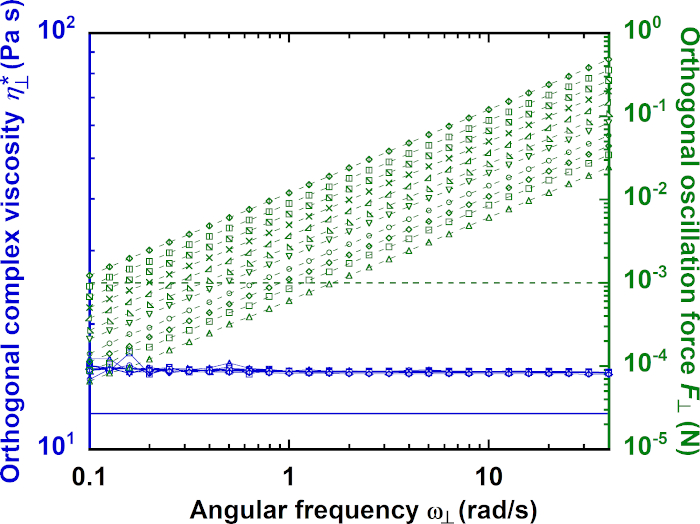Figure 6: Results from orthogonal frequency sweep tests on a 12.2 Pa s viscosity standard. The orthogonal complex viscosity (left y-axis) and oscillation force (right y-axis) are shown as a function of angular frequency. The solid line represents the actual viscosity of the silicone fluid. The dashed line represents the axial oscillation force resolution limit 0.001 N. Different symbols correspond to frequency sweeps at different orthogonal strains. For the oscillation force data, from bottom to top: orthogonal strain (%) = (0.5, 0.7, 0.8, 1.1, 1.6, 2.0, 2.8, 3.9, 5.2, 7.0, and 9.4) %. Please click here to view a larger version of this figure.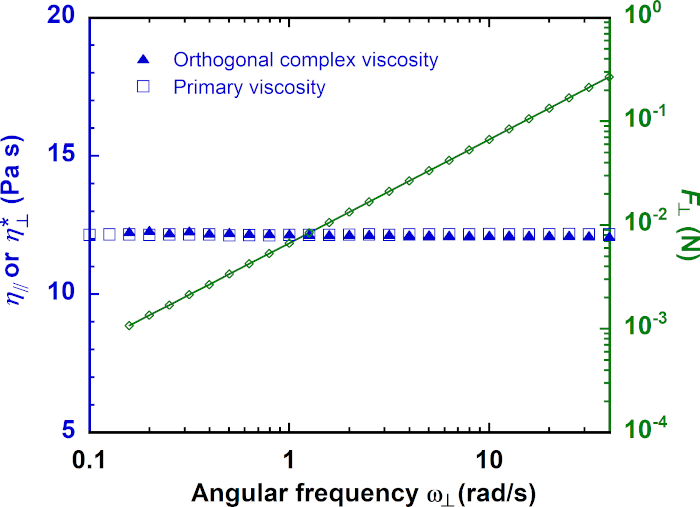Figure 7: Results from orthogonal superposition measurement on a 12.2 Pa s viscosity standard using the calibrated end-effect factors. The test is performed at a shear rate of 1.0 s−1 in the primary angular direction and an oscillatory shear strain of 5.2 % in the orthogonal direction. The orthogonal complex viscosity and primary viscosity (left y-axis) and oscillation force (right y-axis) are shown as a function of angular frequency. Please click here to view a larger version of this figure.

 Parameter description Specifications Minimum transducer torque in steady shear 0.1 µN m Maximum transducer torque 200 mN m Torque resolution 1 nN m Normal/axial force range 0.001 N to 20 N Angular velocity range 10−6 rad s−1 to 300 rad s−1 Minimum force in oscillation (OSP mode) 0.001 N Minimum displacement in oscillation (OSP mode) 0.5 µm Maximum displacement in oscillation (OSP mode) 50 µm Displacement resolution (OSP mode) 10 nm Axial frequency range (OSP mode) 6.28 × 10−5 rad s−1 to 100 rad s−1 APS temperature range −10 °C to 150 °C

Table 1: Specifications of the rheometer and the Advanced Peltier System.

 Parameters in geometry setup Inscribed abbreviation Dimension (mm) Symbol in stress constants Inside cup diameter CID 27.733 2R1 Inside bob diameter ID 28.578 2R2 Outside bob diameter OD 32.997 2R3 Outside cup diameter COD 33.996 2R4 Immersed height (cup height) CH 43.651 h Inner cylinder height 51.651 l

Table 2: The dimensions for the orthogonal double wall concentric cylinder used in the geometry setup as stated by the manufacturer.

 End-effect Factor 1.17 Orthogonal End-effect Factor 0.79 Stress Constant 6541.69 Pa N−1 m−1 Strain Constant 33.4326 rad-1 Stress Constant (Linear) 93.5575 Pa N−1 Strain Constant (Linear) 2136.55 m−1

Table 3: Geometry constants for the 0.5 mm OSP cell. The values of end-effect factor and orthogonal end-effect factor are obtained after calibration.

### Discussion

In this protocol, we present a detailed experimental procedure for performing viscosity calibration measurements using Newtonian fluids for a commercial orthogonal superposition rheology technique with a double-wall concentric cylinder geometry. The calibration factors, i.e., the primary end-effect factor CL and the orthogonal end-effect factor CLo, are determined independently by conducting steady shear rate sweep and orthogonal frequency sweep tests. After obtaining the end factors, a verification test is performed to check the calibration results. The verification test is an orthogonal frequency sweep test superimposed on the primary steady shear, so that the steady-shear viscosity and orthogonal complex viscosity are measured simultaneously. This contrasts with the calibration experiments where each individual test is conducted in the absence of the flow in the orthogonal direction. While this entire procedure is readily comprehensible and adoptable, there are several important steps in the protocol where users should proceed with purpose and care.

First and foremost is proper sample loading. A general rule is to keep the fluid level slightly above the lower rim of the upper opening on the bob, whether the test material is handled by a spatula or a volume adjustable pipette. Keep in mind that the loading process may require lengthy wait times to achieve the desired fluid level (Figure 2). Careful loading of the test material and controlling of the instrument stage are required to avoid the entrapment of air bubbles. By visual inspection of the wetted fluid contact line on the bob (Figure 3), the fluid height in the OSP geometry can be estimated. While the bob is in the up position, it is also important to check if the lower rim of the upper opening on the bob is completely wetted. This step is critical to maintain a fixed bob effective length, or a fixed nominal shear surface, which is helpful to reduce the bob end effects.

We recommended that users use Newtonian liquids with viscosities similar to the liquids for their application needs and perform the calibration measurements reported in this study. The example shown in the present paper is a 12.2 Pa s silicone liquid. The measurement range (i.e., shear rate and angular frequency) (Figure 5 and Figure 6) used for this liquid is based on the instrument limitations (Table 1) and other measurement artifacts, for example, the instrument and fluid inertia. We have reported the appropriate shear rate and orthogonal frequency ranges for Newtonian standards with viscosities ranging from 0.01 Pa s to 331 Pa s in previous work18. Briefly, for the steady shear, the applicable shear rate range is constrained by the transducer torque limits. For the orthogonal shear, the suitable frequency window is subjected to the axial force range, gap width, and fluid properties. Specifically, measurements should be conducted within the gap loading limit that arises from shear wave propagation in viscoelastic fluids19. Understanding the measurement limitations and artifacts are important to avoid any misinterpretation of experimental data20.

We define unity (1.00) as the uncorrected values for the primary end-effect factor CL,uncorr and orthogonal end-effect factor CLo,uncorr to perform the viscosity calibration runs. In fact, the initial values entered for the calibration experiments do not affect the determination of the calibrated end factors. According to Equations 7 and 8, both CL,uncorr and CLo,uncorr act as scale factors for the calculations of CL,corr and CLo,corr. To put it another way, the raw measurement signals (in Equations 3 and 5), i.e., torque M, velocity Ω, orthogonal oscillation force F, displacement θ, and frequency ω, do not depend on the end factor settings in the rheometer software. Regardless, we choose to use 1.00 in the geometry constant settings, simply for the ease of analysis, such that we can find the amount of correction needed by the viscosity outputs from the software in a straightforward manner, as well as discern whether it is overestimation or underestimation if no correction is applied. In both directions, without correction, the measured viscosity overestimates the actual viscosity, as indicated by a greater than unity value for the end-effect factor (1.17) and a less than unity value (0.79) of the orthogonal end-effect factor (Table 2).

The goal of the present paper is to provide visual demonstration of the experimental procedure for the calibration of end-effect factors using Newtonian viscosity standards. For detailed results and analysis of the sources of error for this commercial OSP technique, the readers should refer to our previous publication18. In that work, we performed computational fluid dynamics (CFD) simulations to visualize the velocity, pressure, and the shear rate fields within the entire OSP geometry. The overestimation of primary viscosity is due to a higher average shear rate in the double gap; and the overestimation of the orthogonal viscosity is attributed to the pressure forces on the bob ends in addition to a higher shear rate in the double gap. In addition, error comparisons were discussed among different instruments and between the two commercially available gap size geometries (viz., 0.5 mm and 1.0 mm). We strongly recommend that users determine the end-effect correction factors for their own instrument and geometry, because the actual corrections are material-dependent and will vary among instruments and geometries. The protocol presented in this work is critical to support the growing interest from academic and industrial users that want to apply this technique. Suitable end-effect factors should be applied to obtain correct results, otherwise the errors are appreciable.

The present calibration procedures are carried out for Newtonian fluids, which suggest that the corrections for non-Newtonian fluids could be even larger due to a more complicated flow field within the OSP geometry. As the measurement reliability for non-Newtonian fluids by OSP remains a general concern among the rheology community, future studies will focus on the quantification of end effects and other detrimental effects on the experimental error for non-Newtonian fluids. Understanding the correction related to Newtonian fluid viscosity measurements and the flow field non-idealities within the complicated OSP geometry is the first step for the application of the OSP technique. The protocol presented in this paper paves the way for future investigation on non-Newtonian fluids in order to avoid artifacts and experimental error bias for OSP research.

### Disclosures

The full description of the procedures used in this paper requires the identification of certain commercial products and their suppliers. The inclusion of such information should in no way be construed as indicating that such products or suppliers are endorsed by NIST or are recommended by NIST or that they are necessarily the best materials, instruments, software or suppliers for the purposes described.

### Acknowledgments

Ran Tao would like to thank funding from the National Institute of Standards and Technology, U.S. Department of Commerce under grant 70NANB15H112. Funding for Aaron M. Forster was provided through congressional appropriations to the National Institute of Standards and Technology.

### Materials

 Name Company Catalog Number Comments Advanced Peltier System TA Instruments 402500.901 Enviromental control device ARES-G2 Rheometer TA Instruments 401000.501 Rheometer Brookfield Silicone Fluid, 12500cP AMTEK Brookfield 12500 cps Viscosity standard liquid OSP Slotted Bob, 33 mm TA Instruments 402796.902 Bob, upper geometry OSP Slotted Double Gap Cup, 34 mm TA Instruments 402782.901 Double wall cup, lower geometry Pipette (1 – 10 mL) Eppendorf 3120000089 To load test materials Pipette (100 – 1,000 µL) Eppendorf 3123000063 To load test materials Pipette Tips (0.5 – 10 mL) Eppendorf 022492098 To load test materials Pipette Tips (50 – 1,000 µL) Eppendorf 022491555 To load test materials Spatula VWR 82027-532 To load test materials TRIOS TA Instruments v4.3.1.39215 Rheometer software

Video Coming Soon
DOIX